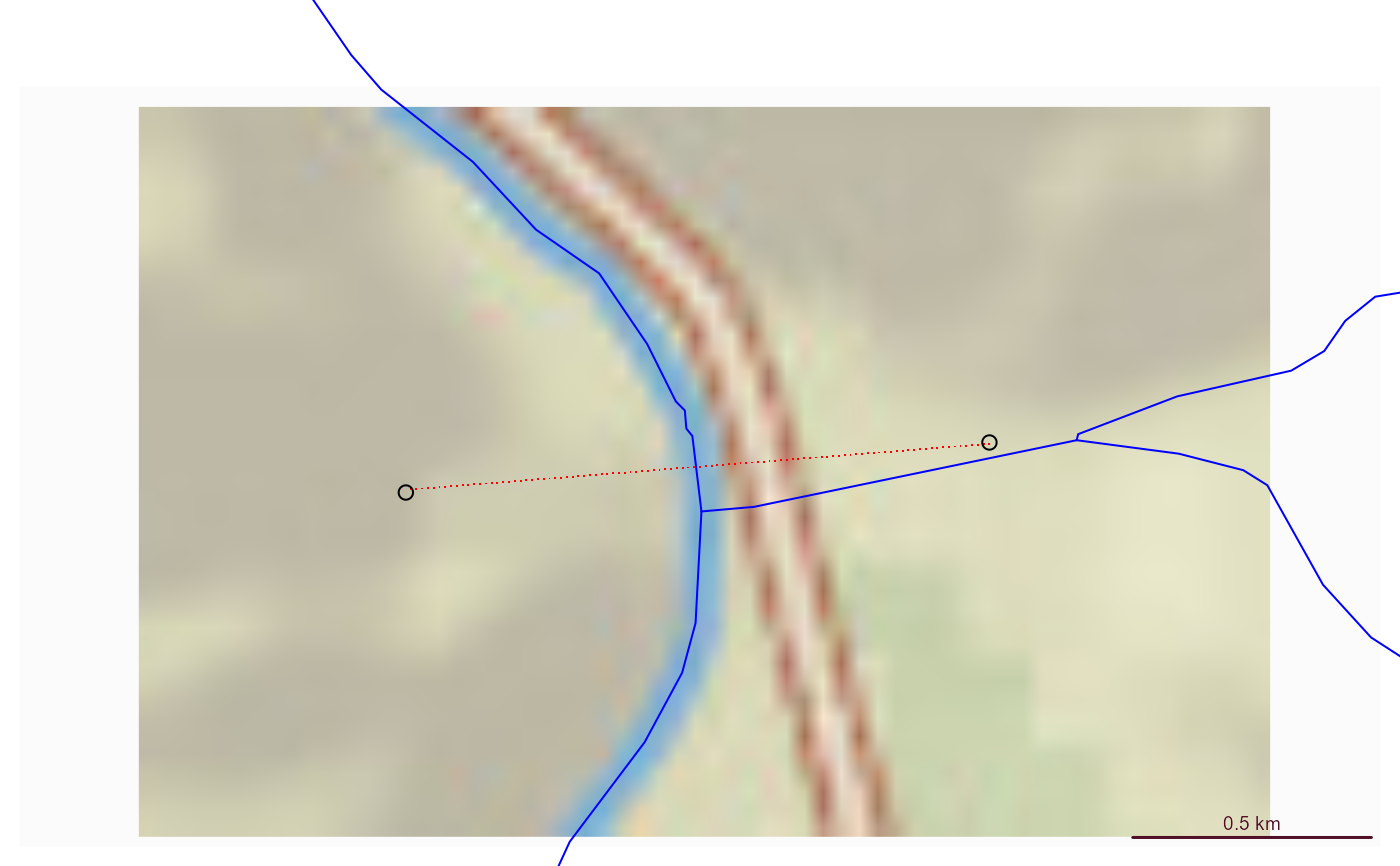Uses a cross section retrieval web services to retrieve a cross section between two endpoints.

get_xs_points(point1, point2, num_pts, res = 1)

## Arguments

point1

sfc POINT including crs as created by: sf::st_sfc(sf::st_point(.. ,..), crs)

point2

sfc POINT including crs.

num_pts

numeric number of points to retrieve along the cross section.

res

integer resolution of 3D Elevation Program data to request. Must be on of: 1, 3, 5, 10, 30, 60.

## Value

sf data.frame containing points retrieved.

## Examples

# \donttest{
point1 <- sf::st_sfc(sf::st_point(x = c(-105.9667, 36.17602)), crs = 4326)
point2 <- sf::st_sfc(sf::st_point(x = c(-105.97768, 36.17526)), crs = 4326)

(xs <- get_xs_points(point1, point2, 100))
#> Simple feature collection with 101 features and 4 fields
#> Geometry type: POINT
#> Dimension:     XY
#> Bounding box:  xmin: -105.9776 ymin: 36.17531 xmax: -105.9667 ymax: 36.176
#> Geodetic CRS:  WGS 84
#> # A tibble: 101 × 5
#>    id    spatial_ref elevation_m distance_m             geometry
#>    <chr>       <int>       <dbl>      <dbl>          <POINT [°]>
#>  1 0               0       1779.       0      (-105.9667 36.176)
#>  2 1               0       1778.       9.74 (-105.9668 36.17599)
#>  3 2               0       1778.      19.5  (-105.9669 36.17599)
#>  4 3               0       1778.      29.2   (-105.967 36.17598)
#>  5 4               0       1776.      39.0  (-105.9671 36.17597)
#>  6 5               0       1776.      48.7  (-105.9672 36.17597)
#>  7 6               0       1776.      58.4  (-105.9674 36.17596)
#>  8 7               0       1775.      68.2  (-105.9675 36.17595)
#>  9 8               0       1775.      77.9  (-105.9676 36.17594)
#> 10 9               0       1774.      87.6  (-105.9677 36.17594)
#> # ℹ 91 more rows

if(inherits(xs, "sf")) {

bbox <- sf::st_bbox(xs) + c(-0.005, -0.005, 0.005, 0.005)

nhdplusTools::plot_nhdplus(bbox = bbox, cache_data = FALSE)

plot(sf::st_transform(sf::st_geometry(xs), 3857), pch = ".", add = TRUE, col = "red")
plot(sf::st_transform(sf::st_sfc(point1, crs = 4326), 3857), add = TRUE)
plot(sf::st_transform(sf::st_sfc(point2, crs = 4326), 3857), add = TRUE)

plot(xs$distance_m, xs$elevation_m)
}
#> Spherical geometry (s2) switched off
#> Found invalid geometry, attempting to fix.
#> although coordinates are longitude/latitude, st_intersects assumes that they
#> are planar
#> Spherical geometry (s2) switched on
#> Spherical geometry (s2) switched off
#> although coordinates are longitude/latitude, st_intersects assumes that they
#> are planar
#> Spherical geometry (s2) switched on
#> Spherical geometry (s2) switched off
#> Warning: No nhdarea features found
#> Spherical geometry (s2) switched on
#> Spherical geometry (s2) switched off
#> Warning: No waterbodies features found
#> Spherical geometry (s2) switched on
#> Spherical geometry (s2) switched off
#> Warning: No nonnetwork features found
#> Spherical geometry (s2) switched on
#> Zoom set to: 12# }